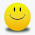Latest Articles

# Squaring of Numbers using Base 50 - Simple and Effective

Info Post
We have seen on our previous post about Squaring of numbers below and above the base of powers of 10.Now using the same technique, we can apply them for numbers in half and in quarters.Let me briefly explain the halfs.

Case 1: Numbers greater than 50
Case 2: Numbers lesser than 50

Example for case 1 :
56 2 = ?
Normal Base = 100 .
For 56 , nearer base is 50 which means [100/2 = 50 ]

Our BASE= 50
Halving the Base = (50/2)=25 ---(number)

Surplus = (50 + 6 ) = 6
Add number + surplus = ( 25 + 6 ) =31 --- (1)
Squaring Surplus = 36 --- (2)

Final answer = (1) | (2) = 31 | 36
= 3136
Therefore  56 2  = 3136
Example for case 2 :
442 = ?
Normal Base = 100 .
For 44 , nearer base is 50 which means [100/2 = 50 ]
Our BASE= 50
Halving the Base = (50/2)=25 ---(number)

Deficit = ( 50 - 44 ) = 6
Add number - deficit = ( 25 -6 ) =19 ---(1)
Squaring deficit = 36 ---(2)

Final answer = (1) | (2) = 19 | 36
= 1936
Therefore  442   = 1936

Share:

1.Really interesting! I love maths, so this was an eye-opener for me! You have a nice blog.... following it now.

Do stop by my blog sometime :) ..... M * U * S * I * N * G * S

Thank You for visiting Momscribe.com !